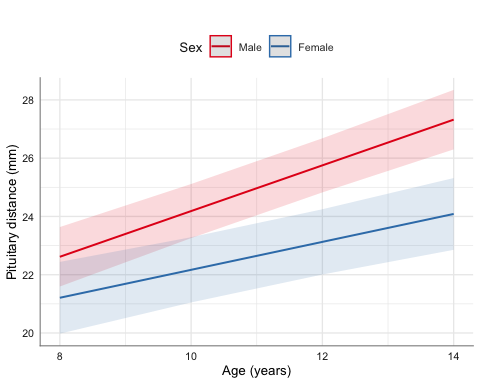# 1 Introduction

The aim of this vignette is to illustrate the use/functionality of the glm_coef function. glm_coef can be used to display model coefficients with confidence intervals and p-values. The advantages and limitations of glm_coef are:

1. Recognises the main models used in epidemiology/public health.
2. Automatically back-transforms estimates and confidence intervals, when the model requires it.
3. Can use robust standard errors for the calculation of confidence intervals.
• Standard errors are used by default.
• The use of standard errors is restricted by the following classes of objects (models): gee, glm and survreg.
4. Can display nice labels for the names of the parameters.
5. Returns a data frame that can be modified and/or exported as tables for publications (with further editing).

We start by loading relevant packages and setting the theme for the plots (as suggested in the Template of this package):

library(broom)
library(car)
library(Hmisc)
library(MASS)
library(kableExtra)
library(tidyverse)
library(mosaic)
library(latex2exp)
library(moonBook)
library(pubh)
library(sjlabelled)
library(sjPlot)

theme_set(sjPlot::theme_sjplot2(base_size = 10))
theme_update(legend.position = "top")
options(knitr.table.format = "pandoc")

# 2 Multiple Linear Regression

For continuous outcomes there is no need of exponentiating the results unless the outcome was fitted in the log-scale. In our first example we want to estimate the effect of smoking and race on the birth weight of babies.

We can generate factors and assign labels in the same pipe stream:

data(birthwt)
birthwt <- birthwt %>%
mutate(
smoke = factor(smoke, labels = c("Non-smoker", "Smoker")),
race = factor(race, labels = c("White", "African American", "Other"))
) %>%
var_labels(
bwt = 'Birth weight (g)',
smoke = 'Smoking status',
race = 'Race'
)

Is good to start with some basic descriptive statistics, so we can compare the birth weight between groups.

birthwt %>%
group_by(race, smoke) %>%
summarise(
n = n(),
Mean = round(mean(bwt, na.rm = TRUE), 2),
SD = round(sd(bwt, na.rm = TRUE), 2),
Median = round(median(bwt, na.rm = TRUE), 2),
CV = round(rel_dis(bwt), 2)
) %>%
kable(caption = "Descriptive statistics of birth weight (g) by ethnicity
and smoking status.")
Descriptive statistics of birth weight (g) by ethnicity and smoking status.
race smoke n Mean SD Median CV
White Non-smoker 44 3428.75 710.10 3593.0 0.21
White Smoker 52 2826.85 626.47 2775.5 0.22
African American Non-smoker 16 2854.50 621.25 2920.0 0.22
African American Smoker 10 2504.00 637.06 2381.0 0.25
Other Non-smoker 55 2815.78 709.35 2807.0 0.25
Other Smoker 12 2757.17 810.04 3146.5 0.29

From the previous table, the group with the lower birth weight was from babies born from African Americans who were smokers. The highest birth weight was from babies born from White non-smokers.

Another way to compare the means between the groups is with gen_bst_df which estimates means with corresponding bootstrapped CIs.

birthwt %>%
gen_bst_df(bwt ~ race|smoke) %>%
kable(caption = "Mean birth weight (g) by ethnicity
and smoking status with 95% CIs.")
Mean birth weight (g) by ethnicity and smoking status with 95% CIs.
Birth weight (g) LowerCI UpperCI Race Smoking status
3428.75 3213.31 3623.35 White Non-smoker
2826.85 2657.57 3000.47 White Smoker
2854.50 2587.38 3143.32 African American Non-smoker
2504.00 2147.59 2839.87 African American Smoker
2815.78 2628.09 2980.30 Other Non-smoker
2757.17 2279.88 3132.78 Other Smoker

Another approach to tabular analysis is graphical analysis. For this comparison, box-plots would be the way to go, but in health sciences it is more common to see bar charts with error bars.

birthwt %>%
bar_error(bwt ~ race, fill = ~ smoke) %>%
axis_labs() %>%
gf_labs(fill = "Smoking status:")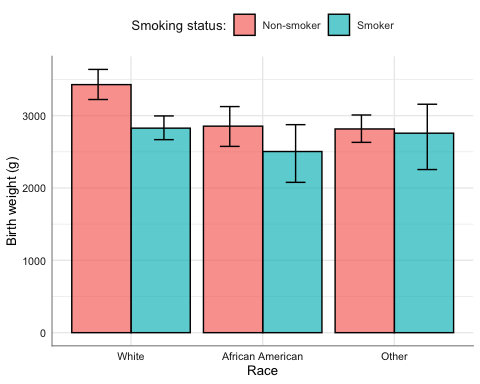We fit a linear model.

model_norm <- lm(bwt ~ smoke + race, data = birthwt)

Note: Model diagnostics are not be discussed in this vignette.

model_norm %>%
Anova()
Anova Table (Type II tests)

Response: bwt
Sum Sq  Df F value    Pr(>F)
smoke      7322575   1 15.4588 0.0001191 ***
race       8712354   2  9.1964 0.0001557 ***
Residuals 87631356 185
---
Signif. codes:  0 '***' 0.001 '**' 0.01 '*' 0.05 '.' 0.1 ' ' 1
model_norm %>%
summary()

Call:
lm(formula = bwt ~ smoke + race, data = birthwt)

Residuals:
Min       1Q   Median       3Q      Max
-2313.95  -440.22    15.78   492.14  1655.05

Coefficients:
Estimate Std. Error t value Pr(>|t|)
(Intercept)           3334.95      91.78  36.338  < 2e-16 ***
smokeSmoker           -428.73     109.04  -3.932 0.000119 ***
raceAfrican American  -450.36     153.12  -2.941 0.003687 **
raceOther             -452.88     116.48  -3.888 0.000141 ***
---
Signif. codes:  0 '***' 0.001 '**' 0.01 '*' 0.05 '.' 0.1 ' ' 1

Residual standard error: 688.2 on 185 degrees of freedom
Multiple R-squared:  0.1234,    Adjusted R-squared:  0.1092
F-statistic: 8.683 on 3 and 185 DF,  p-value: 2.027e-05

Table of coefficients:

model_norm %>%
glm_coef()
Coefficient Pr(>|t|)
(Intercept)          3334.95 (3036.11, 3633.78)  < 0.001
smokeSmoker          -428.73 (-667.02, -190.44)  < 0.001
raceAfrican American  -450.36 (-750.31, -150.4)    0.003
raceOther            -452.88 (-775.17, -130.58)    0.006

Once we know the order in which the parameters are displayed, we can add labels to our final table:

Note: Compare results using naive and robust standard errors.

model_norm %>%
glm_coef(se_rob = FALSE, labels = c("Constant",
"Smoker - No smoker",
"African American - White",
"Other - White")) %>%
kable(caption = "Table of coefficients using naive standard errors.",
align = 'r')
Table of coefficients using naive standard errors.
Coefficient Pr(>|t|)
Constant 3334.95 (3153.89, 3516.01) < 0.001
Smoker - No smoker -428.73 (-643.86, -213.6) < 0.001
African American - White -450.36 (-752.45, -148.27) 0.004
Other - White -452.88 (-682.67, -223.08) < 0.001
model_norm %>%
glm_coef(labels = c("Constant",
"Smoker - No smoker",
"African American - White",
"Other - White")) %>%
kable(caption = "Table of coefficients using robust standard errors.")
Table of coefficients using robust standard errors.
Coefficient Pr(>|t|)
Constant 3334.95 (3036.11, 3633.78) < 0.001
Smoker - No smoker -428.73 (-667.02, -190.44) < 0.001
African American - White -450.36 (-750.31, -150.4) 0.003
Other - White -452.88 (-775.17, -130.58) 0.006

The function glance from the broom package allow us to have a quick look at statistics related with the model.

model_norm %>% glance %>% round(digits = 2)
# A tibble: 1 x 11
r.squared adj.r.squared sigma statistic p.value    df logLik   AIC   BIC
<dbl>         <dbl> <dbl>     <dbl>   <dbl> <dbl>  <dbl> <dbl> <dbl>
1      0.12          0.11  688.      8.68       0     4 -1501. 3012. 3028.
# … with 2 more variables: deviance <dbl>, df.residual <dbl>

To construct the effect plot, we can use plot_model from the sjPlot package. The advantage of plot_model is that recognises labelled data and uses that information for annotating the plots.

plot_model(model_norm, "pred", terms = ~race|smoke, dot.size = 2, title = "")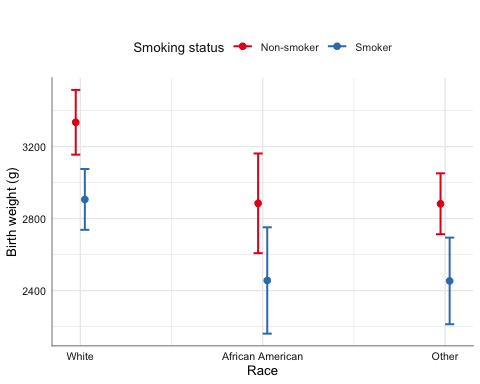When the explanatory variables are categorical, another option is emmip from the emmeans package. We can include CIs in emmip but as estimates are connected, the resulting plots look more messy, so I recommend emmip to look at the trace.

emmip(model_norm, smoke ~ race) %>%
gf_labs(y = get_label(birthwtbwt), x = "", col = "Smoking status")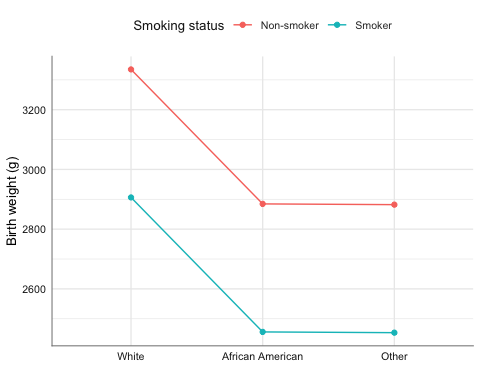# 3 Logistic Regression For logistic regression we are interested in the odds ratios. We will look at the effect of amount of fibre intake on the development of coronary heart disease. data(diet, package = "Epi") diet <- diet %>% mutate( chd = factor(chd, labels = c("No CHD", "CHD")) ) %>% var_labels( chd = "Coronary Heart Disease", fibre = "Fibre intake (10 g/day)" ) We start with descriptive statistics: diet %>% estat(fibre ~ chd) %>% kable Coronary Heart Disease N Min. Max. Mean Median SD CV Fibre intake (10 g/day) No CHD 288 0.60 5.35 1.75 1.69 0.58 0.33 CHD 45 0.76 2.43 1.49 1.51 0.40 0.27 It is standard to plot the dependent variable in the $$y$$-axis, so in this case, we can use horizontal box-plots. diet %>% gf_boxploth(chd ~ fibre, fill = "indianred3", alpha = 0.7) %>% axis_labs()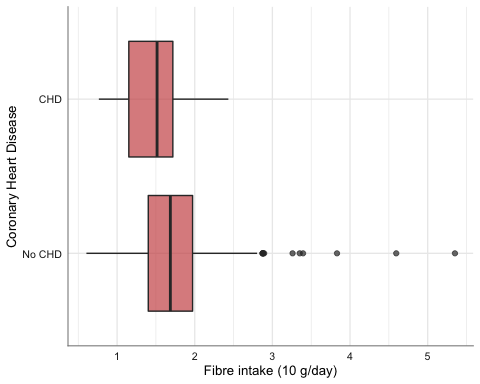We fit a linear logistic model: model_binom <- glm(chd ~ fibre, data = diet, family = binomial) model_binom %>% glm_coef(labels = c("Constant", "Fibre intake (g/day)")) %>% kable(caption = "Parameter estimates from logistic regression.", align = "r") Parameter estimates from logistic regression. Odds ratio Pr(>|z|) Constant 0.95 (0.3, 3.01) 0.934 Fibre intake (g/day) 0.33 (0.16, 0.67) 0.002 model_binom %>% glance %>% round(digits = 2) # A tibble: 1 x 7 null.deviance df.null logLik AIC BIC deviance df.residual <dbl> <dbl> <dbl> <dbl> <dbl> <dbl> <dbl> 1 264. 332 -127. 258. 265. 254. 331 Effect plot: plot_model(model_binom, "pred", terms = "fibre [all]", title = "")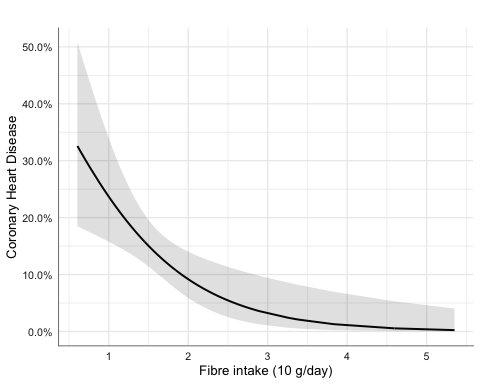## 3.1 Matched Case-Control Studies: Conditional Logistic Regression We will look at a matched case-control study on the effect of oestrogen use and history of gall bladder disease on the development of endometrial cancer. data(bdendo, package = "Epi") bdendo <- bdendo %>% mutate( cancer = factor(d, labels = c('Control', 'Case')), gall = factor(gall, labels = c("No GBD", "GBD")), est = factor(est, labels = c("No oestrogen", "Oestrogen")) ) %>% var_labels( cancer = 'Endometrial cancer', gall = 'Gall bladder disease', est = 'Oestrogen' ) We start with descriptive statistics: mytable(cancer ~ est + gall, data = bdendo, show.total = TRUE) %>% mytable2df() %>% kable() Endometrial cancer Control Case Total p (N=252) (N=63) (N=315) Oestrogen 0.000 - No oestrogen 125 (49.6%) 7 (11.1%) 132 (41.9%) - Oestrogen 127 (50.4%) 56 (88.9%) 183 (58.1%) Gall bladder disease 0.001 - No GBD 228 (90.5%) 46 (73.0%) 274 (87.0%) - GBD 24 ( 9.5%) 17 (27.0%) 41 (13.0%) We fit the conditional logistic model: model_clogit <- clogit(cancer == 'Case' ~ est * gall + strata(set), data = bdendo) model_clogit %>% glm_coef(labels = c("Oestrogen/No oestrogen", "GBD/No GBD", "Oestrogen:GBD Interaction")) %>% kable() Odds ratio Pr(>|z|) Oestrogen/No oestrogen 14.88 (4.49, 49.36) < 0.001 GBD/No GBD 18.07 (3.2, 102.01) 0.001 Oestrogen:GBD Interaction 0.13 (0.02, 0.9) 0.039 model_clogit %>% glance %>% round(digits = 2) # A tibble: 1 x 15 n nevent statistic.log p.value.log statistic.sc p.value.sc statistic.wald <dbl> <dbl> <dbl> <dbl> <dbl> <dbl> <dbl> 1 315 63 49.3 0 40.6 0 25.1 # … with 8 more variables: p.value.wald <dbl>, r.squared <dbl>, # r.squared.max <dbl>, concordance <dbl>, std.error.concordance <dbl>, # logLik <dbl>, AIC <dbl>, BIC <dbl> Creating data frame needed to construct the effect plot: require(ggeffects) bdendo_pred <- ggemmeans(model_clogit, terms = c('gall', 'est')) Effect plot: bdendo_pred %>% gf_pointrange(predicted + conf.low + conf.high ~ x|group, col = ~ x) %>% gf_labs(y = "P(cancer)", x = "", col = get_label(bdendogall))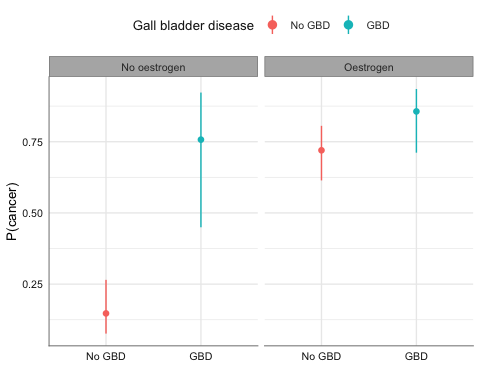## 3.2 Ordinal Logistic Regression

We use data about house satisfaction.

library(ordinal, warn.conflicts = FALSE)
data(housing)
housing <- housing %>%
var_labels(
Sat = "Satisfaction",
Infl = "Perceived influence",
Type = "Type of rental",
Cont = "Contact"
)

We fit the ordinal logistic model:

model_clm <- clm(Sat ~ Infl + Type + Cont, weights = Freq, data = housing)
labs_ord <- c("Constant: Low/Medium satisfaction",
"Constant: Medium/High satisfaction",
"Perceived influence: Medium/Low",
"Perceived influence: High/Low",
"Accommodation: Apartment/Tower",
"Accommodation: Atrium/Tower",
"Accommodation: Terrace/Tower",
"Afforded: High/Low")
kable(glm_coef(model_clm, labels = labs_ord),
caption = "Parameter estimates on satisfaction of householders.")
Parameter estimates on satisfaction of householders.
Ordinal OR Pr(>|Z|)
Constant: Low/Medium satisfaction 0.61 (0.48, 0.78) < 0.001
Constant: Medium/High satisfaction 2 (1.56, 2.55) < 0.001
Perceived influence: Medium/Low 1.76 (1.44, 2.16) < 0.001
Perceived influence: High/Low 3.63 (2.83, 4.66) < 0.001
Accommodation: Apartment/Tower 0.56 (0.45, 0.71) < 0.001
Accommodation: Atrium/Tower 0.69 (0.51, 0.94) 0.018
Accommodation: Terrace/Tower 0.34 (0.25, 0.45) < 0.001
Afforded: High/Low 1.43 (1.19, 1.73) < 0.001
model_clm %>% glance %>% round(digits = 2)
# A tibble: 1 x 5
edf logLik   AIC   BIC df.residual
<dbl>  <dbl> <dbl> <dbl>       <dbl>
1     8 -1740. 3495. 3539.        1673

Effect plot:

plot_model(model_clm, dot.size = 1, title = "")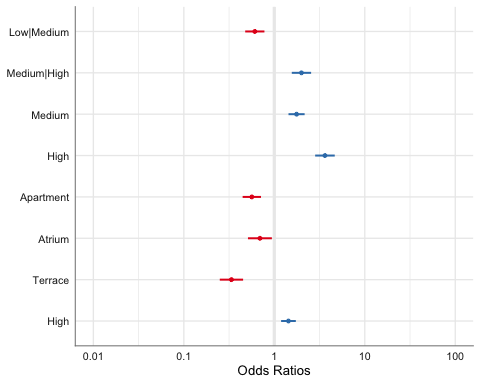plot_model(model_clm, type = "pred", terms = c("Infl", "Cont"),
dot.size = 1, title = "") %>%
gf_theme(axis.text.x = element_text(angle = 45, hjust = 1))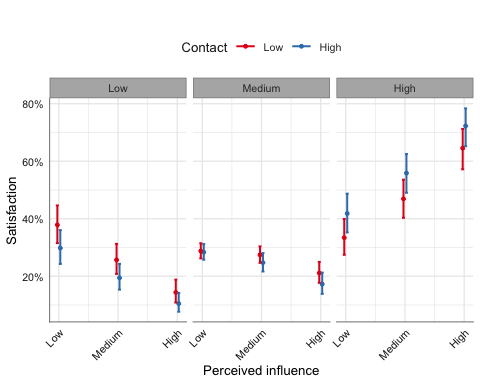plot_model(model_clm, type = "pred", terms = c("Infl", "Type"),
dot.size = 1, title = "") %>%
gf_theme(axis.text.x = element_text(angle = 45, hjust = 1))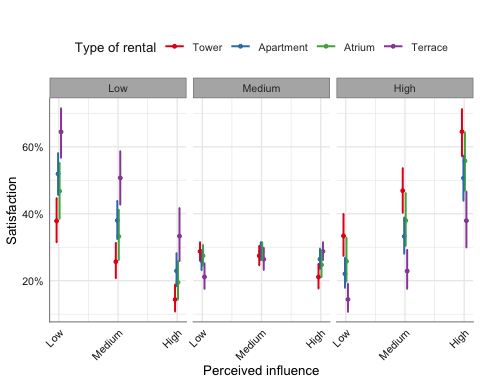emmip(model_clm, Infl ~ Type |Cont) %>%
gf_labs(x = "Type of rental", col = "Perceived influence")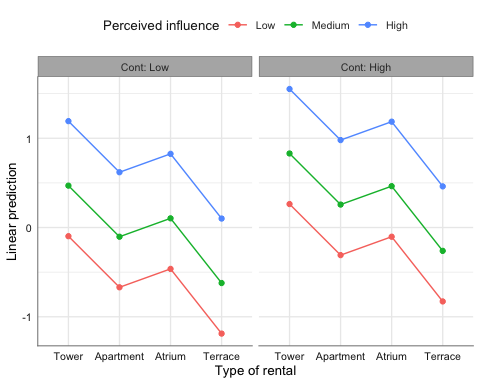Note: In the previous table parameter estimates and confidence intervals for Perceived influence and Accommodation were not adjusted for multiple comparisons.

# 4 Poisson Regression

For Poisson regression we are interested in incidence rate ratios. We will look at the effect of sex, ethnicity and age group on number of absent days from school in a year.

data(quine)
levels(quine$Eth) <- c("Aboriginal", "White") levels(quine$Sex) <- c("Female", "Male")
quine <- quine %>%
var_labels(
Days = "Number of absent days",
Eth = "Ethnicity",
Age = "Age group"
)

Descriptive statistics:

quine %>%
group_by(Eth, Sex, Age) %>%
summarise(
n = n(),
Mean = round(mean(Days, na.rm = TRUE), 2),
SD = round(sd(Days, na.rm = TRUE), 2),
Median = round(median(Days, na.rm = TRUE), 2)
) %>%
kable()
Eth Sex Age n Mean SD Median
Aboriginal Female F0 5 17.60 17.37 11
Aboriginal Female F1 15 18.87 16.33 13
Aboriginal Female F2 9 32.56 27.31 20
Aboriginal Female F3 9 14.56 14.85 10
Aboriginal Male F0 8 11.50 7.23 12
Aboriginal Male F1 5 9.60 4.51 7
Aboriginal Male F2 11 30.91 17.81 32
Aboriginal Male F3 7 27.14 10.37 28
White Female F0 5 19.80 9.68 20
White Female F1 17 7.76 6.48 6
White Female F2 10 5.70 4.97 4
White Female F3 10 13.50 11.49 12
White Male F0 9 13.56 20.85 7
White Male F1 9 5.56 5.39 5
White Male F2 10 15.20 12.88 12
White Male F3 7 27.29 22.93 27

We start by fitting a standard Poisson linear regression model:

model_pois <- glm(Days ~ Eth + Sex + Age, family = poisson, data = quine)
glm_coef(model_pois) %>% kable
Rate ratio Pr(>|z|)
(Intercept) 17.66 (10.66, 29.24) < 0.001
EthWhite 0.59 (0.39, 0.88) 0.01
SexMale 1.11 (0.77, 1.6) 0.57
AgeF1 0.8 (0.42, 1.5) 0.482
AgeF2 1.42 (0.85, 2.36) 0.181
AgeF3 1.35 (0.77, 2.34) 0.292
model_pois %>% glance %>% round(digits = 2)
# A tibble: 1 x 7
null.deviance df.null logLik   AIC   BIC deviance df.residual
<dbl>   <dbl>  <dbl> <dbl> <dbl>    <dbl>       <dbl>
1         2074.     145 -1165. 2343. 2361.    1742.         140

## 4.1 Negative-binomial

The assumption is that the mean is equal than the variance. If that is the case, deviance should be close to the degrees of freedom of the residuals (look at the above output from glance). In other words, the following calculation should be close to 1:

deviance(model_pois) / df.residual(model_pois)
 12.44646

Thus, we have over-dispersion. One option is to use a negative binomial distribution.

model_negbin <- glm.nb(Days ~ Eth + Sex + Age, data = quine)
glm_coef(model_negbin,
labels=c(
"Constant",
"Race: Aboriginal/White",
"Sex: Female/Male",
"F1/Primary",
"F2/Primary",
"F3/Primary")
) %>%
kable()
Rate ratio Pr(>|z|)
Constant 20.24 (12.24, 33.47) < 0.001
Race: Aboriginal/White 0.57 (0.38, 0.84) 0.005
Sex: Female/Male 1.07 (0.74, 1.53) 0.73
F1/Primary 0.69 (0.39, 1.23) 0.212
F2/Primary 1.2 (0.7, 2.03) 0.507
F3/Primary 1.29 (0.73, 2.28) 0.385
model_negbin %>% glance %>% round(digits = 2)
# A tibble: 1 x 7
null.deviance df.null logLik   AIC   BIC deviance df.residual
<dbl>   <dbl>  <dbl> <dbl> <dbl>    <dbl>       <dbl>
1          192.     145  -548. 1110. 1131.     168.         140

Notice that age group is a factor with more than two levels and is significant:

model_negbin %>%
Anova()
Analysis of Deviance Table (Type II tests)

Response: Days
LR Chisq Df Pr(>Chisq)
Eth  12.6562  1  0.0003743 ***
Sex   0.1486  1  0.6998722
Age   9.4844  3  0.0234980 *
---
Signif. codes:  0 '***' 0.001 '**' 0.01 '*' 0.05 '.' 0.1 ' ' 1

Thus, we want to report confidence intervals and $$p$$-values adjusted for multiple comparisons.

Effect plot:

plot_model(model_negbin, type = "pred", terms = c("Age", "Eth"),
dot.size = 1, title = "")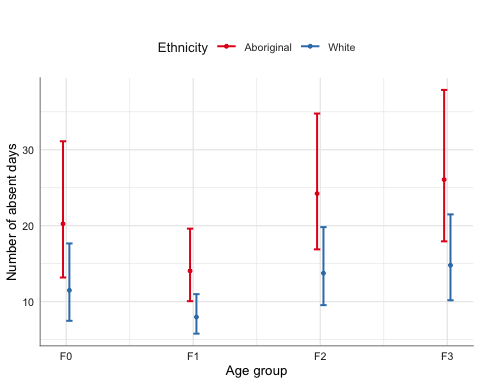emmip(model_negbin, Eth ~ Age|Sex) %>%
gf_labs(y = "Number of absent days", x = "Age group", col = "Ethnicity")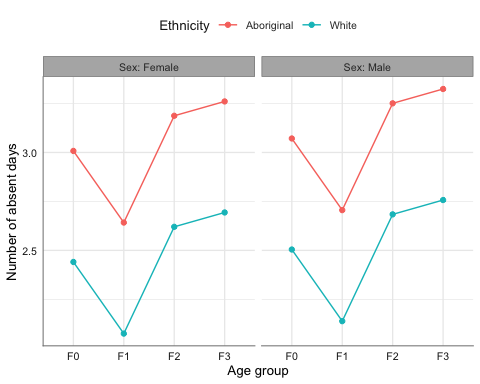## 4.2 Adjusting CIs and p-values for multiple comparisons

multiple(model_negbin, ~ Age|Eth)$df contrast Eth estimate SE df z.ratio p.value 1 F0 - F1 Aboriginal 0.37 0.23 Inf 1.57 0.395 2 F0 - F2 Aboriginal -0.18 0.23 Inf -0.77 0.865 3 F0 - F3 Aboriginal -0.25 0.24 Inf -1.04 0.724 4 F1 - F2 Aboriginal -0.55 0.21 Inf -2.65 0.040 5 F1 - F3 Aboriginal -0.62 0.21 Inf -2.89 0.020 6 F2 - F3 Aboriginal -0.07 0.22 Inf -0.34 0.987 7 F0 - F1 White 0.37 0.23 Inf 1.57 0.395 8 F0 - F2 White -0.18 0.23 Inf -0.77 0.865 9 F0 - F3 White -0.25 0.24 Inf -1.04 0.724 10 F1 - F2 White -0.55 0.21 Inf -2.65 0.040 11 F1 - F3 White -0.62 0.21 Inf -2.89 0.020 12 F2 - F3 White -0.07 0.22 Inf -0.34 0.987 We can see the comparison graphically with: multiple(model_negbin, ~ Age + Sex|Eth)$fig_ci %>%
gf_labs(y = "Age group", x = "Number of absent days")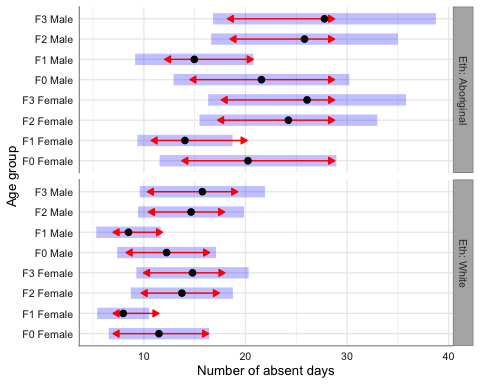# 5 Survival Analysis

We will use an example on the effect of thiotepa versus placebo on the development of bladder cancer.

data(bladder)
mutate(times = stop,
rx = factor(rx, labels=c("Placebo", "Thiotepa"))
) %>%
var_labels(times = "Survival time",
rx = "Treatment")

## 5.1 Parametric method

model_surv <- survreg(Surv(times, event) ~ rx, data = bladder)

Using robust standard errors (default):

model_surv %>%
glm_coef(labels = c("Treatment: Thiotepa/Placebo", "Scale")) %>%
kable()
Survival time ratio Pr(>|z|)
Treatment: Thiotepa/Placebo 1.64 (0.89, 3.04) 0.116
Scale 1 (0.85, 1.18) 0.992

In this example the scale parameter is not statistically different from one, meaning hazard is constant and thus, we can use the exponential distribution:

model_exp <- survreg(Surv(times, event) ~ rx, data = bladder, dist = "exponential")
model_exp %>%
glm_coef(labels = c("Treatment: Thiotepa/Placebo")) %>%
kable()
Survival time ratio Pr(>|z|)
Treatment: Thiotepa/Placebo 1.64 (0.85, 3.16) 0.139
model_exp %>% glance %>% round(digits = 2)
# A tibble: 1 x 8
iter    df statistic p.value logLik   AIC   BIC df.residual
<dbl> <dbl>     <dbl>   <dbl>  <dbl> <dbl> <dbl>       <dbl>
1     5     2      6.54    0.01  -594. 1192. 1199.         338

Interpretation: Patients receiving Thiotepa live on average 64% more than those in the Placebo group.

Using naive standard errors:

model_exp %>%
glm_coef(labels = c("Treatment: Thiotepa/Placebo"), se_rob = FALSE) %>%
kable()
Survival time ratio Pr(>|z|)
Treatment: Thiotepa/Placebo 1.64 (1.11, 2.41) 0.012
plot_model(model_exp, type = "pred", terms = ~ rx, dot.size = 1, title = "") %>%
gf_labs(y = "Survival time", x = "Treatment", title = "")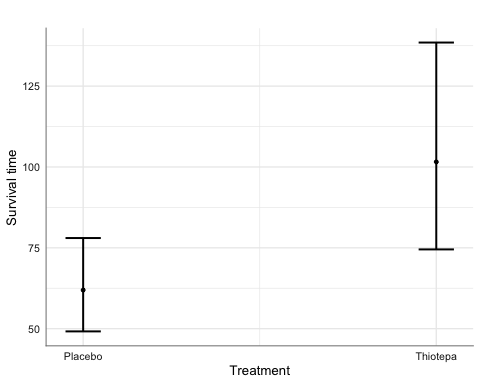## 5.2 Cox proportional hazards regression

model_cox <-  coxph(Surv(times, event) ~ rx, data = bladder)
model_cox %>%
glm_coef(labels = c("Treatment: Thiotepa/Placebo")) %>%
kable()
Hazard ratio Pr(>|z|)
Treatment: Thiotepa/Placebo 0.64 (0.44, 0.94) 0.024
model_cox %>% glance %>% round(digits = 2)
# A tibble: 1 x 15
n nevent statistic.log p.value.log statistic.sc p.value.sc statistic.wald
<dbl>  <dbl>         <dbl>       <dbl>        <dbl>      <dbl>          <dbl>
1   340    112          5.24        0.02         5.14       0.02           5.06
# … with 8 more variables: p.value.wald <dbl>, r.squared <dbl>,
#   r.squared.max <dbl>, concordance <dbl>, std.error.concordance <dbl>,
#   logLik <dbl>, AIC <dbl>, BIC <dbl>

Interpretation: Patients receiving Thiotepa are 64% less likely of dying than those in the Placebo group.

plot_model(model_cox, type = "pred", terms = ~ rx, dot.size = 1,
title = "") %>%
gf_labs(x = "Treatment", title = "")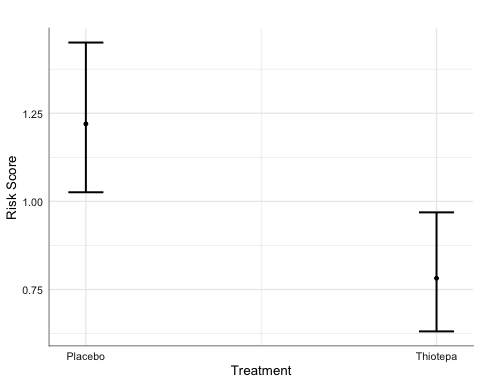# 6 Mixed Linear Regression Models

## 6.1 Continuous outcomes

We look at the relationship between sex and age on the distance from the pituitary to the pterygomaxillary fissure (mm).

library(nlme)
data(Orthodont)
Orthodont <- Orthodont %>%
var_labels(
distance = "Pituitary distance (mm)",
age = "Age (years)"
)

We fit the model:

model_lme <- lme(distance ~ Sex * I(age - mean(age, na.rm = TRUE)), random = ~ 1|Subject,
method = "ML", data = Orthodont)
model_lme %>%
glm_coef(labels = c("Constant", "Sex: female-male", "Age (years)",
"Sex:Age interaction")) %>%
kable()
Coefficient Pr(>|t|)
Constant 24.97 (24.03, 25.9) < 0.001
Sex: female-male -2.32 (-3.78, -0.86) 0.005
Age (years) 0.78 (0.63, 0.94) < 0.001
Sex:Age interaction -0.3 (-0.54, -0.07) 0.015
model_lme %>% glance
# A tibble: 1 x 5
sigma logLik   AIC   BIC deviance
<dbl>  <dbl> <dbl> <dbl> <lgl>
1  1.37  -214.  441.  457. NA      

Effect plot:

plot_model(model_lme, type = "pred", terms = age ~ Sex) %>%
gf_labs(y = get_label(Orthodont\$distance), x = "Age (years)", title = "")